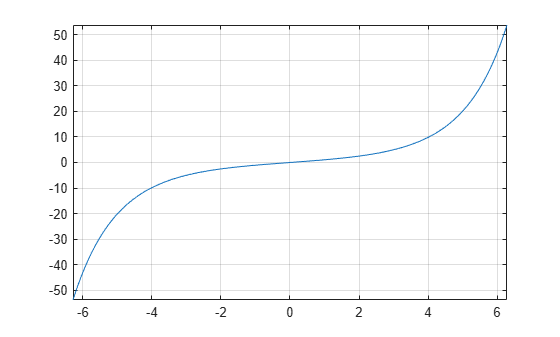# sinhint

Hyperbolic sine integral function

## Syntax

``sinhint(X)``

## Description

example

````sinhint(X)` returns the hyperbolic sine integral function of `X`.```

## Examples

### Hyperbolic Sine Integral Function for Numeric and Symbolic Arguments

Depending on its arguments, `sinhint` returns floating-point or exact symbolic results.

Compute the hyperbolic sine integral function for these numbers. Because these numbers are not symbolic objects, `sinhint` returns floating-point results.

`A = sinhint([-pi, -1, 0, pi/2, 2*pi])`
```A = -5.4696 -1.0573 0 1.8027 53.7368```

Compute the hyperbolic sine integral function for the numbers converted to symbolic objects. For many symbolic (exact) numbers, `sinhint` returns unresolved symbolic calls.

`symA = sinhint(sym([-pi, -1, 0, pi/2, 2*pi]))`
```symA = [ -sinhint(pi), -sinhint(1), 0, sinhint(pi/2), sinhint(2*pi)]```

Use `vpa` to approximate symbolic results with floating-point numbers:

`vpa(symA)`
```ans = [ -5.4696403451153421506369580091277,... -1.0572508753757285145718423548959,... 0,... 1.802743198288293882089794577617,... 53.736750620859153990408011863262]```

### Plot Hyperbolic Sine Integral Function

Plot the hyperbolic sine integral function on the interval from `-2*pi` to `2*pi`.

```syms x fplot(sinhint(x),[-2*pi 2*pi]) grid on```### Handle Expressions Containing Hyperbolic Sine Integral Function

Many functions, such as `diff`, `int`, and `taylor`, can handle expressions containing `sinhint`.

Find the first and second derivatives of the hyperbolic sine integral function:

```syms x diff(sinhint(x), x) diff(sinhint(x), x, x)```
```ans = sinh(x)/x ans = cosh(x)/x - sinh(x)/x^2```

Find the indefinite integral of the hyperbolic sine integral function:

`int(sinhint(x), x)`
```ans = x*sinhint(x) - cosh(x)```

Find the Taylor series expansion of `sinhint(x)`:

`taylor(sinhint(x), x)`
```ans = x^5/600 + x^3/18 + x```

## Input Arguments

collapse all

Input, specified as a symbolic number, variable, expression, or function, or as a vector or matrix of symbolic numbers, variables, expressions, or functions.

collapse all

### Hyperbolic Sine Integral Function

The hyperbolic sine integral function is defined as follows:

`$\text{Shi}\left(x\right)=\underset{0}{\overset{x}{\int }}\frac{\mathrm{sinh}\left(t\right)}{t}dt$`

 Gautschi, W. and W. F. Cahill. “Exponential Integral and Related Functions.” Handbook of Mathematical Functions with Formulas, Graphs, and Mathematical Tables. (M. Abramowitz and I. A. Stegun, eds.). New York: Dover, 1972.

## Support

#### Mathematical Modeling with Symbolic Math Toolbox

Get examples and videos